Adrian Colyer’s The Morning Paper impresses me, so I’m copying it.

Model-Agnostic Meta-Learning for Fast Adaptation of Deep Networks

## The Goal

Find a function $$f^*$$ that maximizes performance/minimizes loss on a task. Let’s call it an optimal function. It may not be unique.

In ML, we take a roundabout approach to finding $$f^*$$ by parametrizing the whole problem.

## Lots of Formal Definitions

I’m going to start by not starting with the paper, but with a bunch of definitions. As a mathematician, my process of understanding is to come up with definitions and refine them as I learn more. These are for my own clarification. They were not obvious to me and took embarrassingly long to think of. I made them up. They’re not necessarily the best definitions, and though they may turn out to be lies, I don’t think any of them are misleading.

Feel free to skip them.

### The Correspondence Between Models and Weights

Model
A function $$f$$ meant to approximate $$f^*$$. In math, $$f \approx f^*$$.
Architecture
The functional form of a model. In a neural network, it’s the graph that shows how everything is wired together.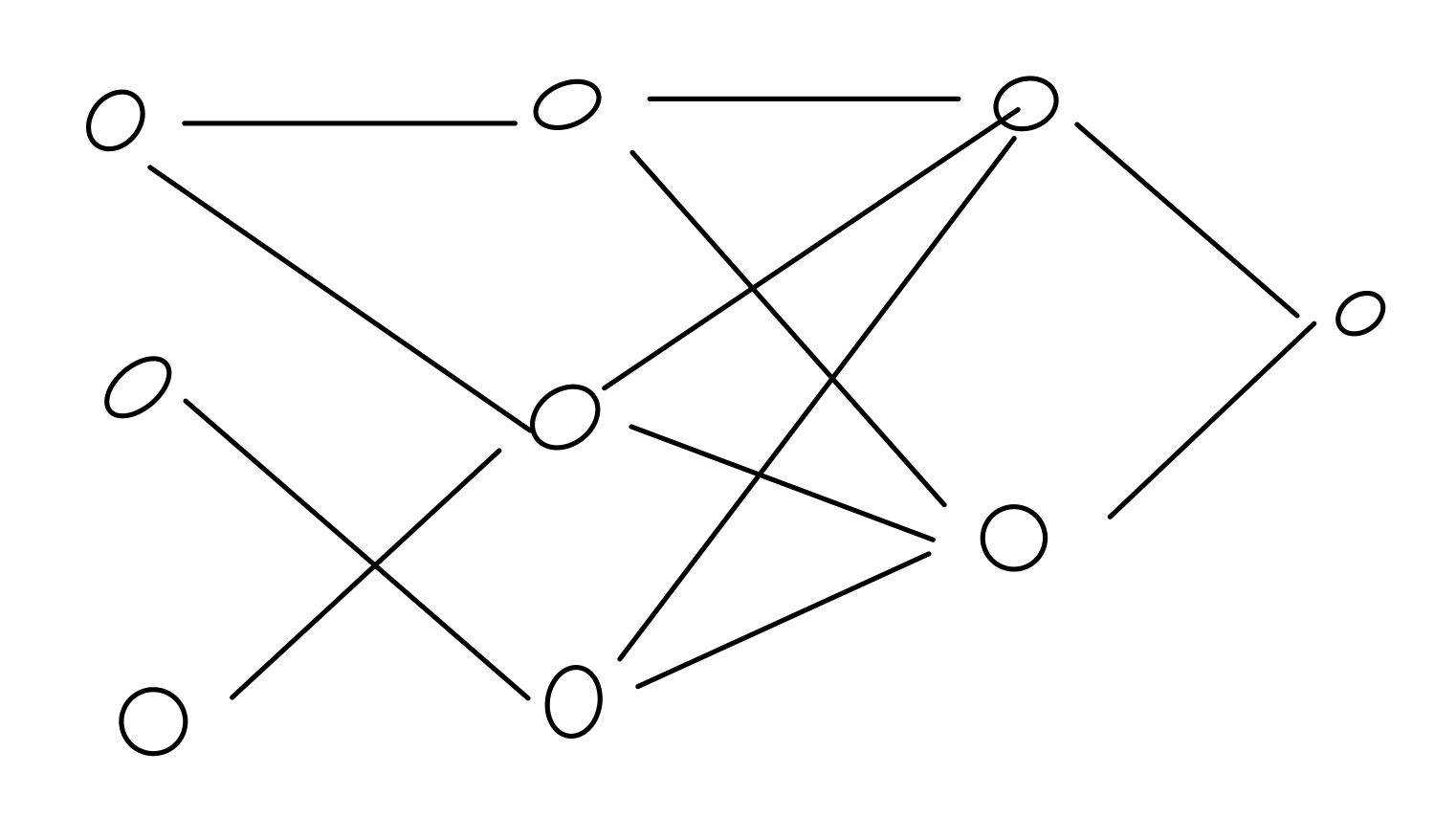Weights
A set of values (usually real numbers) that, combined with an architecture, define a model.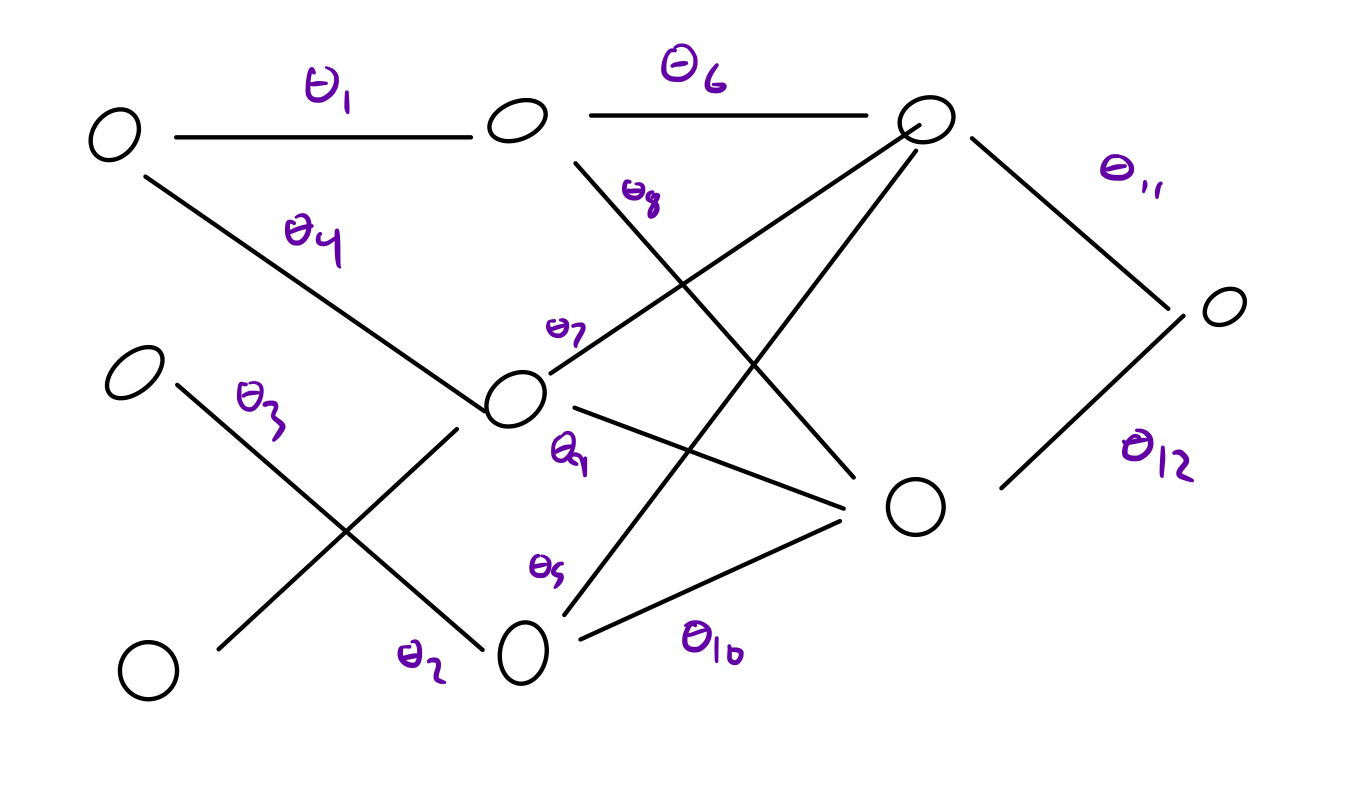Different architectures give different models from identical sets of weights.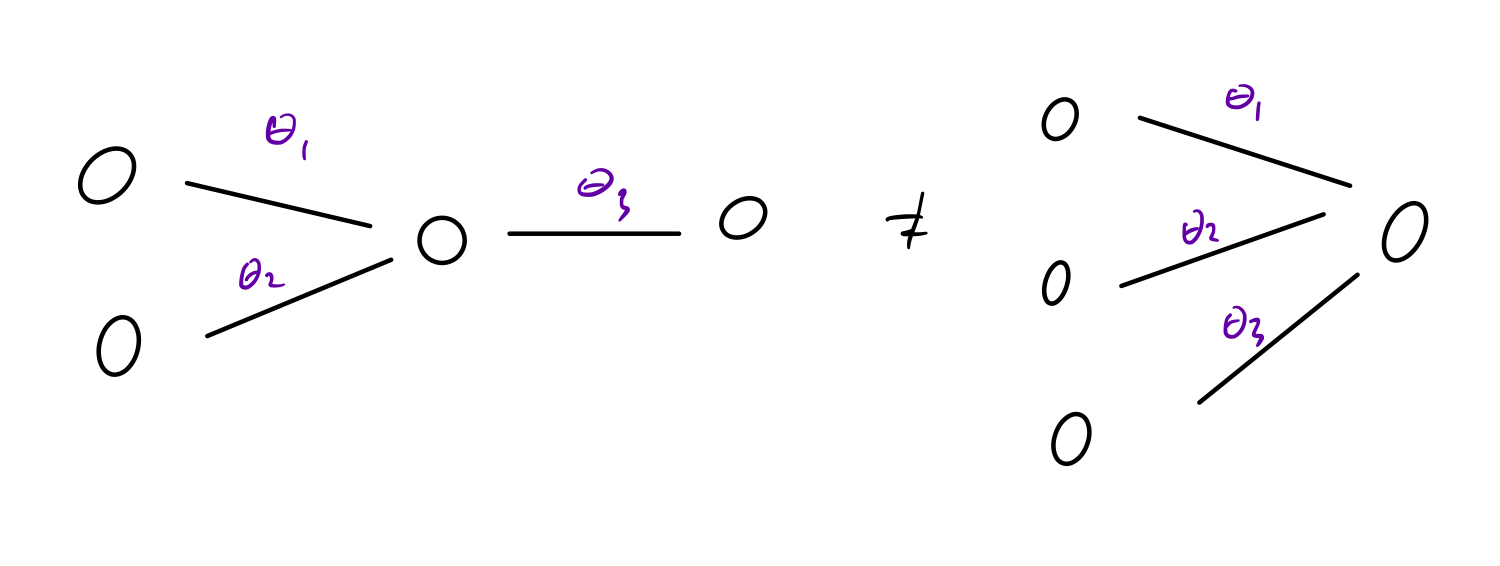The following 2 terms are used in the paper:

Representation
A set of weights with a fixed architecture.
Deep representation
The fixed architecture in question is deep (imagine a neural network with a lot of layers).

Fix an architecture. Then different weights will lead to different models. But not always. Consider a fully connected network, which is invariant to permuting weights.1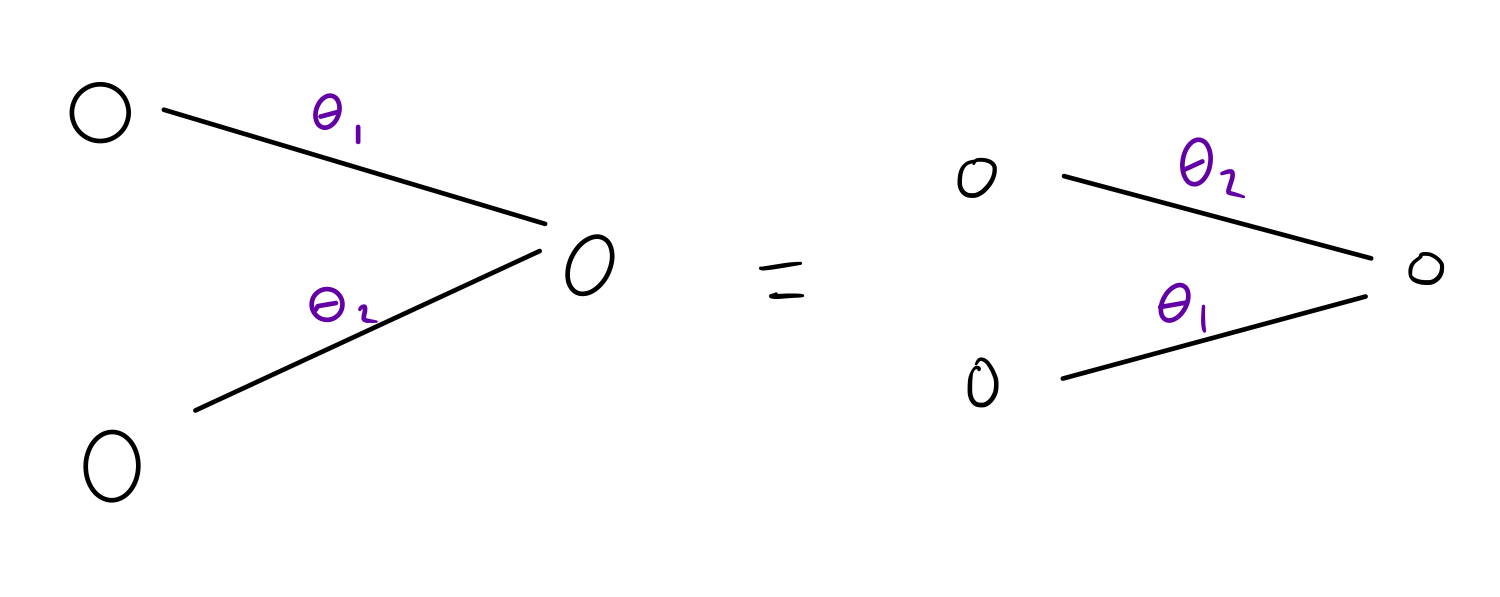Dataset
$$D:= \{(x,y)\}$$, a set of input/output pairs.
Synonym for dataset (for our purposes).
Training
Let $$f_{\theta}$$ be a model $$f$$ parametrized by weights $$\theta$$. Then training is a function from data to new weights $$\theta'$$ with type signature $$D \to \theta' \leftrightarrow f_{\theta'}$$. Basically, we use the data to update the weights.

The upshot of all this is that we can change The Goal from “find an optimal function” to “fix an architecture that’s sufficiently expressive, then find an optimal set of weights for it”. We reduce the problem of finding a function to the problem of finding a good set of numbers. Numbers are easier than functions.

## Learning vs. Meta-Learning

In regular supervised learning, our dataset is generated from some distribution $$p$$.

In meta-learning, $$p$$ itself is generated from a distribution $$P$$, a distribution over distributions (hence the meta).

In the image below, $$P$$ is a zero-mean Gaussian, and sampling from it gives the parameters of the distributions $$p_i$$. Sampling from the $$p_i$$ would give data like the points below.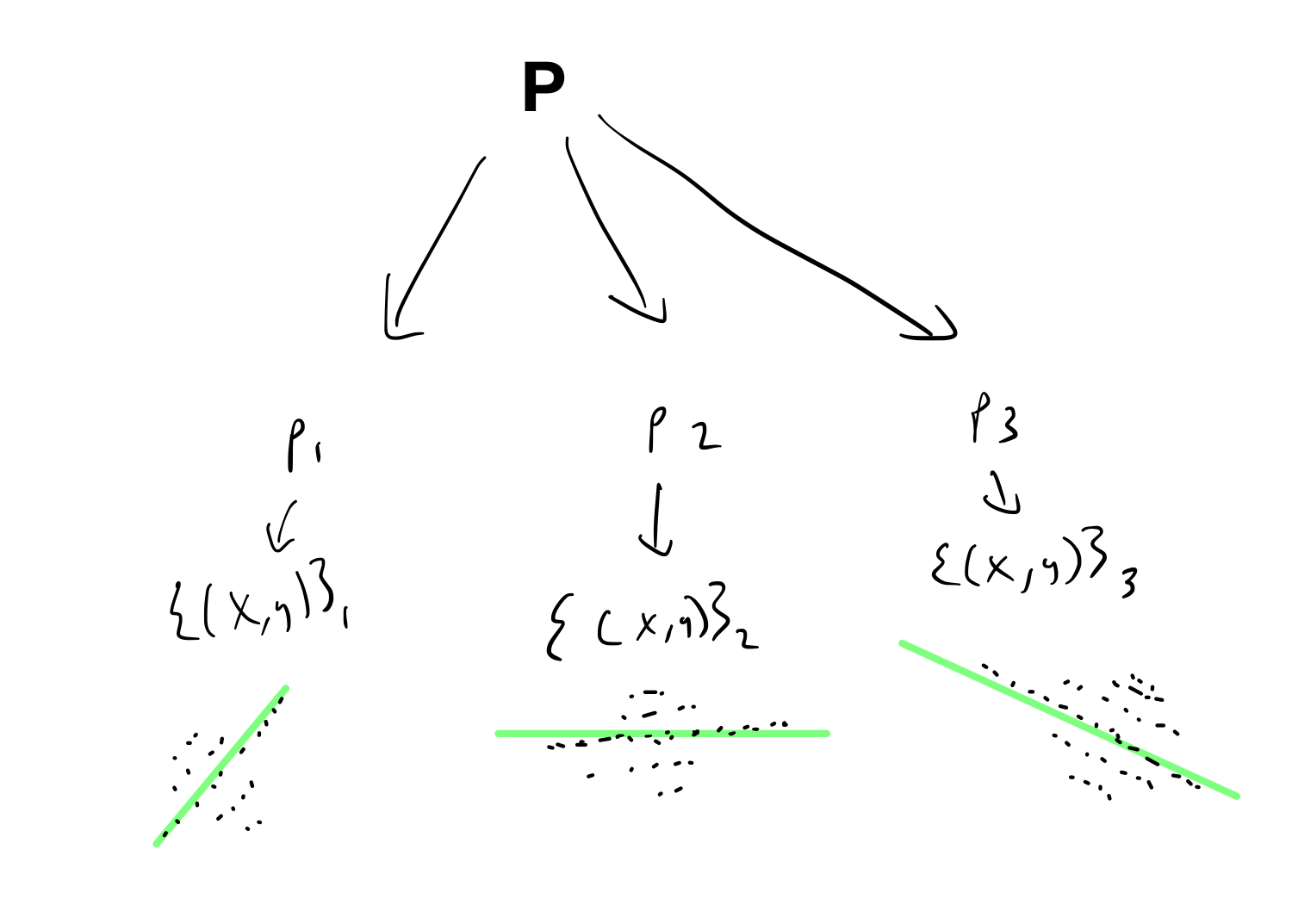Meta-Training
Training a model on a set of datasets, then using the adapted weights to update the orginal set

In other words: in regular learning, we sample data points from a task. In meta-learning, we sample entire tasks.

## Basic Idea

I like MAML because it introduces a principle, and principles are more useful than algorithms. The principle can be used whenever you need to optimize over a class of problems generated from the same distribution. (The paper also has an algorithm.)

The principle is to find weights $$\theta$$ that are close to good weights $$\theta_i$$ for a class of tasks. Since you’re close to good for any given task, you only need a few steps of gradient descent to update $$\theta \to \theta_i$$.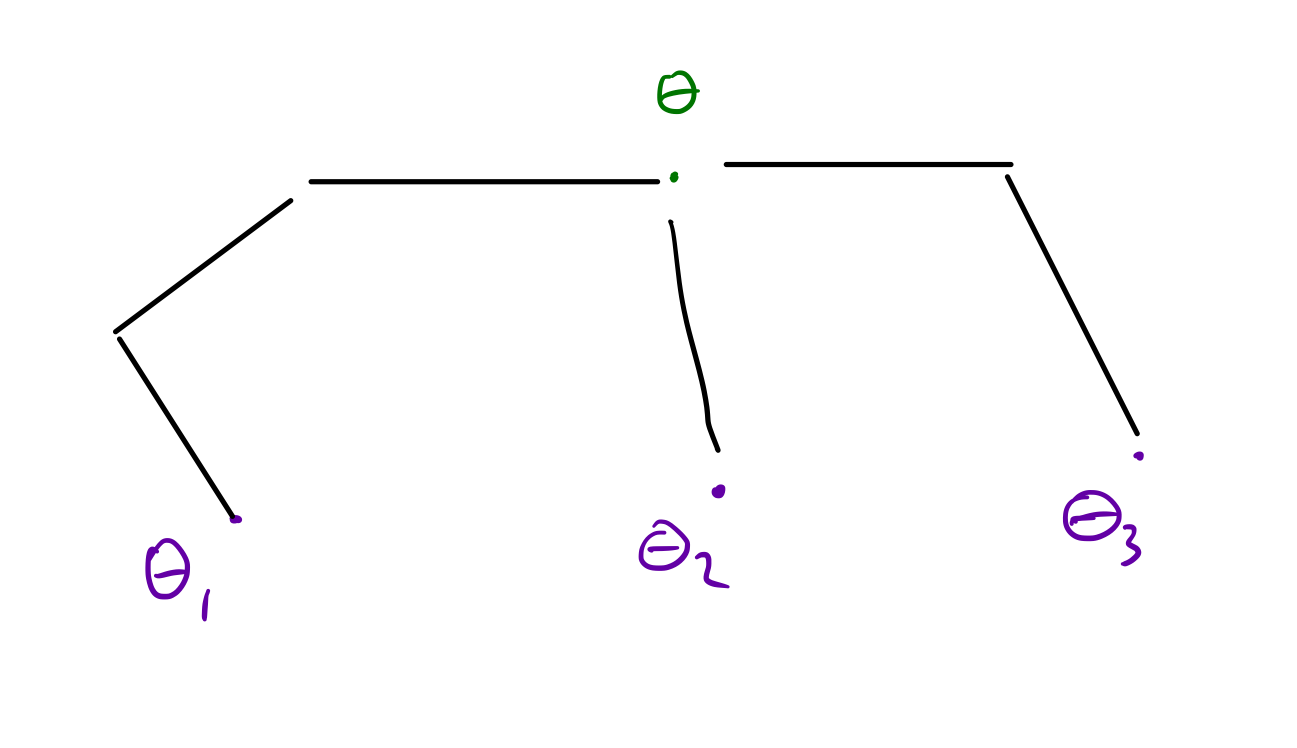Since what we really want is to take few gradient descent steps to adapt to a new task, being close to good sets of weights isn’t enough. We also need to take big gradient steps. In more technical terms, the derivative of the loss function with respect to the weights should have large magnitude, since then small changes in the weights lead to big changes in the loss function.

Basically, this is good initialization and fast fine-tuning on steroids.

## MAML in a Type Signature

$\{D_i\} \to \{\theta_i\} \to \theta$

## Meta-Loss

This is simple. Minimize the sum of losses across tasks of each adapted model $$f_i := f_{\theta_i}$$.

## Sketch of the Algorithm

If you want a proper algorithm, check out the paper. Here’s a sketchy version.

Initialize a starting set of weights $$\theta$$. Given a distribution over tasks $$P$$, sample a minibatch of tasks $$T_i$$. Run supervised learning on each tasks via minibatch SGD. Compute the updated weights $$\theta_i$$ for each task (using $$\theta$$ as your starting weights). Update $$\theta$$ by finding the derivative of the meta-loss function.

Repeat.

## Issues

### Second Derivatives

You’re taking a derivative of a derivative when you differentiate the meta-loss function. Second derivatives (aka Hessians) can be computationally expensive to compute.

On the other hand, Chelsea pointed out that in experiments, computing second derivatives only gave a 33% overhead.

If that’s still too expensive, first-order approximations seem to do fine. The why is not fully known yet, but part of it seems to be that ReLU networks are locally almost linear, so the higher-order terms are near 0.

## Extensions

In the paper, 2 further algorithms are provided for using MAML for few-shot learning and reinforcement learning. They’re mostly the same as the basic sketch given, because MAML is really a generic optimization procedure. I liked the sketches anyway, since the authors have done the hard work and I don’t have to come up with them.

1. In mathematical terms, the map from weights to a model is not injective. For a fixed architecture, it’s also not surjective as there always exist functions that require more hidden units to approximate well. If you let the architecture vary, the fact that neural networks are universal approximators of continuous functions makes it surjective.